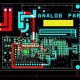# Best Interview Questions and Answer about Transistor

In this tutorial we are going to learn about Best Interview Questions and Answer about Transistor

Question1. What is transistor?

Answer – It is a semiconductor device which is used to amplify or switch electronic signals and electrical power. It has least three terminals for connection to an external circuit.

Question2.  How many types of transistor?

Answer – Two types of transistor like NPN & PNP.

Question3. How types of terminals of transistor?

Answer – Transistor has three terminals like base, emitter and collector.

Question4. How many types of transistor biased?

Answer – Transistor has two types biased – 1. Forward biased and 2. Reverse biased.

Question5. Why use transistor?

Answer – It is use to amplify the signals and switching signals.

Question6. How many junction of transistor?

Answer – Transistor has two PN junctions.

Question7. In PNP transistor, the current carries are?

Answer – Holes are the current carries in PNP transistor.

Question8. Which types of doping in transistor of collector?

Answer – Moderately doping in transistor of collector.

Question9. Which types transistor operation device?

Answer – Transistor is current operated device.

Quetion10. Which types of minority carriers in NPN transistor?

Answer – NPN transistor has holes types of minority carriers.

Question11. What is forward biased?

Answer – When in circuit P side are connected to positive source and N side is connected to negative source.

Question12. What is reverse biased?

Answer – In circuit when P side is connected to negative side and N side is connected to positive side.

Question13. Which types of doping in transistor of emitter?

Answer – Emitter of transistor has heavily doping in transistor.

Question14. What is the input impedance of a transistor?

Answer – Transistor has low input impedance.

Question15. In transistor, Emitter current is equal to?

Answer – In transistor IE = IC+UB

Question16. What is value of ὰ in transistor?

Answer – Less than 1 value of ὰ in transistor.

Question17. What is meaning of FET?

Answer – FET meaning is field effect transistor.

Question18. What is Meaning of BJT?

Answer – BJT is meaning of bipolar transistor.

Question19. Transistor is uni – polar or bi-polar

Answer – Transistor is uni-polar device.

Question20. How many types of transistor region of operation?

Answer – Transistor has four region of operation like – 1. Saturation Region 2.Active Region 3. Inverse Active Region 4. Cut-off Region.## About EEE

We have designing Experience for the last 40 years.